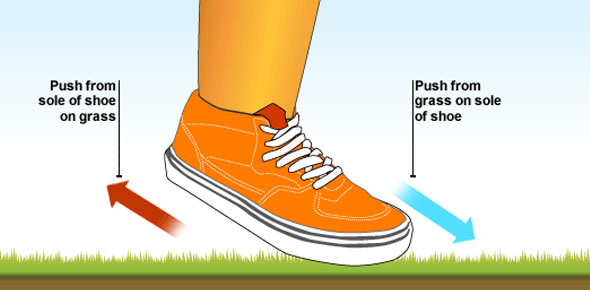# Moving Motion Simulation Part 3

11 Questions | Total Attempts: 195Settings• 1.
Step one:  Make sure that all of moving mans values are set to zero and that the tab is set on "introduction". Step two:  Set the moving mans velocity to 5 m/s and the acceleration to -2 m/s/s Step three:  Press play then press pause when the moving man returns to its starting point.   Question: Which of the following best describe how blue slider (position) moved
• A.

It moved to right at a constant pace

• B.

It moved to left at a constant pace

• C.

It moved to the right then turned around and moved to the left

• D.

It moved to the left then turned around and moved to the right

• E.

It remained stationary

• 2.
The blue slider (position) remained a positive value for the entire time
• A.

True

• B.

False

• 3.
Which of the following best describes the motion of red slider (velocity).
• A.

It remains stationary

• B.

It moves to the left

• C.

It moves to the right

• D.

It moves to the left then to the right

• 4.
The red slider (velocity) remains positive the entire time.
• A.

True

• B.

False

• 5.
At what point does the slider turns from positive to negative.
• A.

When the moving starts

• B.

When the moving man is paused

• C.

When the moving man reaches the apex of his move (maximum distance from the starting point)

• D.

It never turns negative

• 6.
The reason the red slider (velocity) turns from positive to negative is because the moving man changed direction, he quit moving to the right and started moving to the left.
• A.

True

• B.

False

• 7.
How does the green slider (acceleration) behave?
• A.

It moved to the left

• B.

It moves to the right

• C.

It moves to the right then to the left

• D.

It remains stationary

• 8.
Step one:  Click on the tab "chart" set the position to zero the velocity to 5 m/s and velocity to -2 m/s/s  Predict what the position graph will look like
• 9.
Predict what the velocity graph will look like
• 10.
Predict what acceleration graph look like.
• 11.
Press play and pause when the moving man returns to his starting point. Where you predictions correct?  Why does the graphs appear the way they do?
Related TopicsBack to top